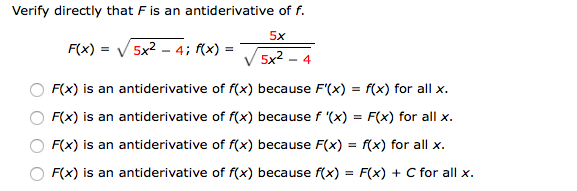Verify directly that F is an antiderivative of f.F(X) = V/ 5x2-4; f(x)--5x2-45xF(x) is an antiderivative of f(x) because F(x) - f(x) for allxF(x) is an antiderivative of f(x) because f '(x) - Fx) for all >xF(x) is an antiderivative of rx) because F(x) = rx) for all x.F(x) is an antiderivative of f(x) because f(x) - F(x) + C for all x

Questionhelp_outlineImage TranscriptioncloseVerify directly that F is an antiderivative of f. F(X) = V/ 5x2-4; f(x)--5x2-4 5x F(x) is an antiderivative of f(x) because F(x) - f(x) for allx F(x) is an antiderivative of f(x) because f '(x) - Fx) for all >x F(x) is an antiderivative of rx) because F(x) = rx) for all x. F(x) is an antiderivative of f(x) because f(x) - F(x) + C for all x fullscreen
Step 1

It is given that,

Step 2

Use chain rule differentiate F(x) with respect to x.

Step 3

From the above calculation it is observed that F’(x) = f(x).

Hence, the option 1 is true, that is, F(x) is an antiderivative of f(x...

Want to see the full answer?

See Solution

Want to see this answer and more?

Our solutions are written by experts, many with advanced degrees, and available 24/7

See Solution
Tagged in

Calculus